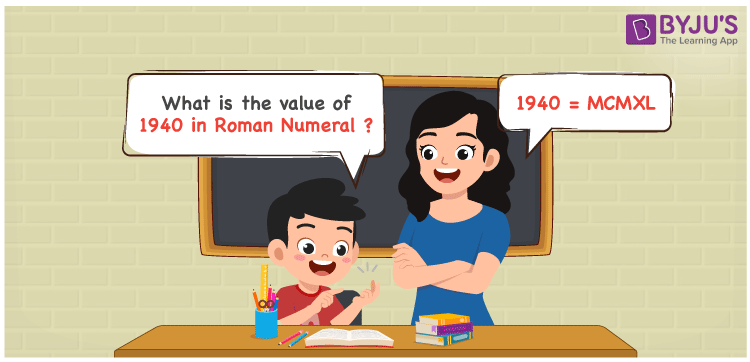# 1940 in Roman Numerals

1940 in Roman Numerals is expressed as MCMXL. Roman Numerals are the letters used by the Romans for the illustration of cardinal numbers. Solving the problems using the PDF of Roman Numerals 1 to 50 on a regular basis helps students to convert the natural numbers 1 to 50 into Roman Numerals effortlessly. Learn the simple way of representing 1940 in Roman Numerals in this article.

 Number Roman Numeral 1940 MCMXL

## How to Write 1940 in Roman Numerals?We can convert the number 1940 into Roman Numerals by using the method given below

• Break the number 1940 into the simplest form
• 1940 = 1000 + (1000 – 100) + (50 – 10)
• Write their respective Roman letter and add/subtract them
• 1940 = M + (M – C) + (L – X) = MCMXL
• Hence the value of 1940 in Roman Numerals is MCMXL

## Video Lesson on Roman Numerals## Related Articles

Roman Numerals

Roman Numerals 1 to 100

Roman Numerals 1 to 1000

Roman Numerals Conversion

L Roman Numeral

Roman Numeral XXXIX

## Frequently Asked Questions on MCMXL Roman Numerals

Q1

### What is 1940 in Roman Numerals?

1940 in Roman Numerals is MCMXL.
Q2

### What is the value of 1000 + 940 in Roman Numerals?

1000 + 940 = 1940. The number 1940 in Roman Numerals is written as MCMXL. Therefore the value of 1000 + 940 in Roman Numerals is MCMXL.
Q3

### What is the value of (100 – 78) + 1940 in Roman Numerals?

(100 – 78) + 1940 = 1962. The number 1962 in Roman Numerals is MCMLXII. Thus the value of (100 – 78) + 1940 in Roman Numerals is MCMLXII.